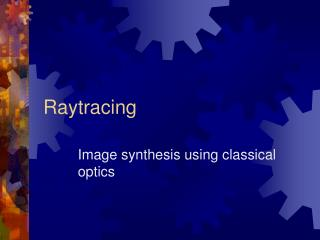DownloadDownload PresentationRaytracing

# Raytracing

Télécharger la présentation## Raytracing

- - - - - - - - - - - - - - - - - - - - - - - - - - - E N D - - - - - - - - - - - - - - - - - - - - - - - - - - -
##### Presentation Transcript

1. Raytracing Image synthesis using classical optics

2. Raytracing • Create an image by following the paths of rays through a scene • “Backward raytracing": traces rays out of the light sources out into the world • “Forward raytracing": traces rays out from the eye (camera) and out into the world and eventually back to the light sources

3. Forward Raytracing • Simplest form (raycasting): • for each pixel on the screen, send a ray out into the world • determine the first surface hit by the ray • perform a lighting calculation at that surface point • set the color of the pixel according to the result of the lighting calculation

4. Raytracing rays from eye screen

5. Rays • A ray is a half-line – it has only one end • It can be written p = o + t*d, t in (0,∞) p is position (vector) o is the origin (vector) d is direction (vector) t is the parameter

6. Ray Intersection • Key computation: intersection of ray with objects • Typically produced by solving an equation: p = f(t) Say the object is defined by an implicit surface, g(x,y,z) = 0 Then g(f(t)) = 0 • Smallest value of t means first intersection

7. Ray Intersection

8. Ray-Sphere Intersection • Implicit equation of sphere: • (x-xc)2 + (y-yc)2 + (z-zc)2– R2 = 0 • Or, (p-pc)•(p-pc) – R2 = 0 • Say ray is given by p(t) = e + t*d • Then (d • d)t2 + 2d•(e - c)t + (e – c)•(e – c) – R2 = 0

9. Other Quadrics • Cylinders, ellipsoids, paraboloids, hyperboloids: all have the property that they are algebraic surfaces of degree 2, aka quadrics • The intersection calculation can be done analytically using the quadratic equation

10. Lighting Calculation • So… we know where the ray hits the surface • But what does the surface look like? • Need to compute lighting at the point of intersection

11. Local Lighting Calculation • Can use 3-term lighting calculation • local illumination • Raytracing allows us to do global illumination • shadows • reflected and refracted light

12. Shadow Rays • Decide if a point is in shadow or not • based on new ray intersection test • Cast ray from intersection point towards light source • If intersection found, point is in shadow • Otherwise, not in shadow

13. Shadow ray Transmitted rays Reflected rays Ray intersection RaytracingTree Resolving a ray intersection can result in multiple additional rays

14. Reflected Rays • Recursive call to raycast: • ray’s color is (1-c) times the color computed at the surface, plus c times the color computed by a new ray heading out of the surface in the reflected direction • May cast multiple reflected rays at slightly different directions • Need to terminate the recursion: • maximum depth • minimum energy carried by the ray

15. Accelerating Intersections • Majority of work in raytracer in computing intersections • Simple-minded method: compute intersections with every object in scene, take smallest parameter • Need to speed this up • bounding geometry • spatial partitioning

16. Raytracingvs Z-Buffer • Initial intersection test is the hard part • Z-buffer still supreme • But, all kinds of effects with same code • transparency, reflection, shadows, caustics • in Z-buffer, bizarre stack of methods • "Embarrassingly parallel": well suited to GPU from process viewpoint – but, recursion not possible on cards